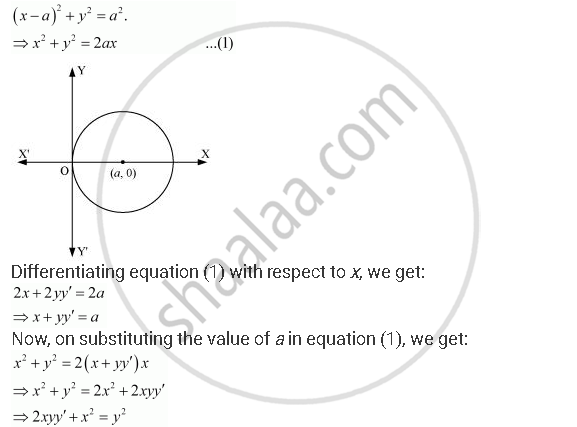Share

# Form the Differential Equation of the Family of Circles Touching the Y-axis at the Origin. - CBSE (Science) Class 12 - Mathematics

ConceptProcedure to Form a Differential Equation that Will Represent a Given Family of Curves

#### Question

Form the differential equation of the family of circles touching the y-axis at the origin.

#### Solution

The centre of the circle touching the y-axis at origin lies on the x-axis.

Let (a, 0) be the centre of the circle.

Since it touches the y-axis at origin, its radius is a.

Now, the equation of the circle with centre (a, 0) and radius (a) isThis is the required differential equation.

Is there an error in this question or solution?

#### APPEARS IN

NCERT Solution for Mathematics Textbook for Class 12 (2018 to Current)
Chapter 9: Differential Equations
Q: 6 | Page no. 391
Solution Form the Differential Equation of the Family of Circles Touching the Y-axis at the Origin. Concept: Procedure to Form a Differential Equation that Will Represent a Given Family of Curves.
S